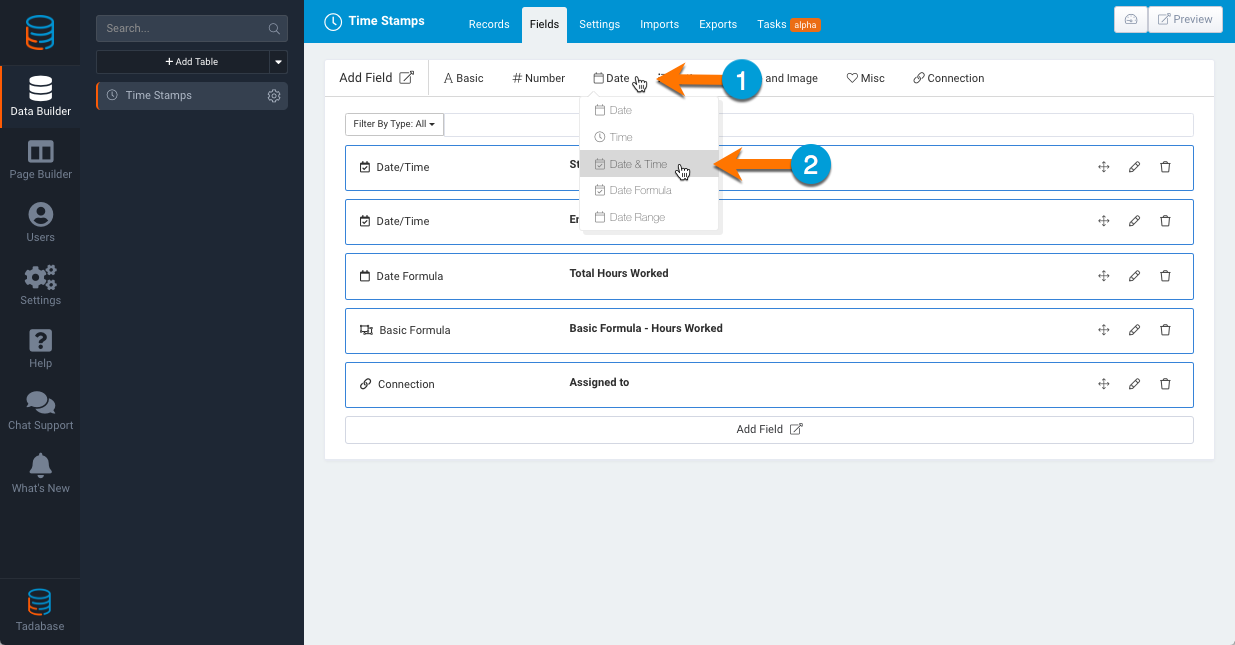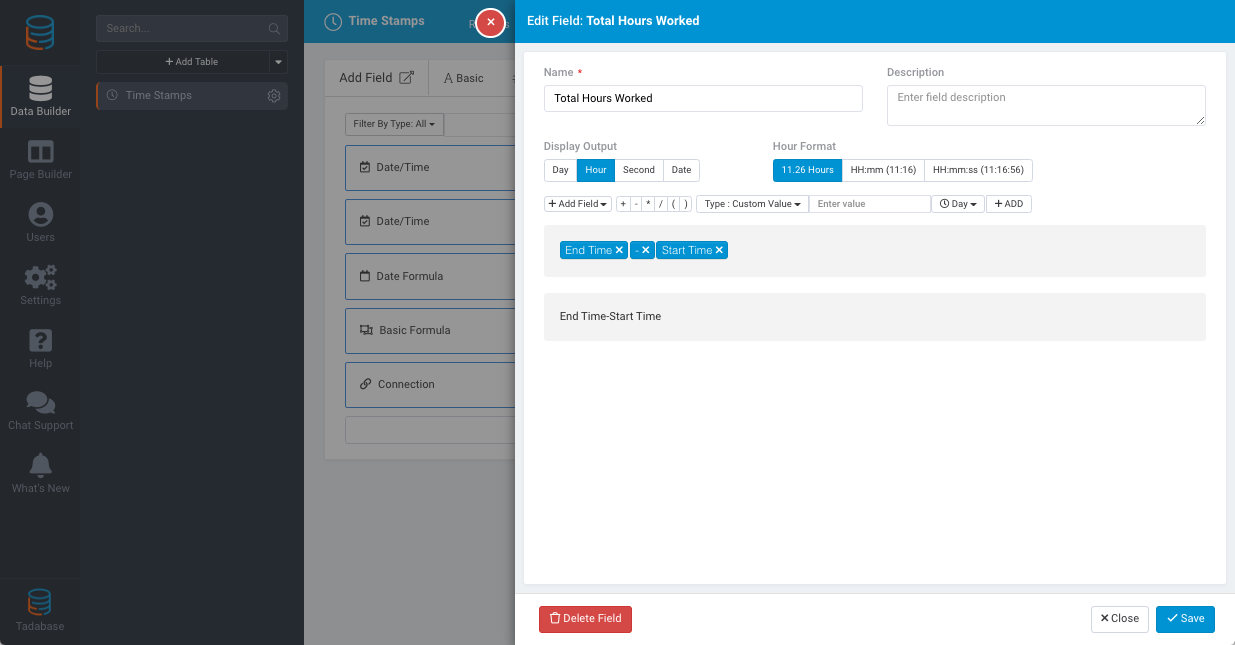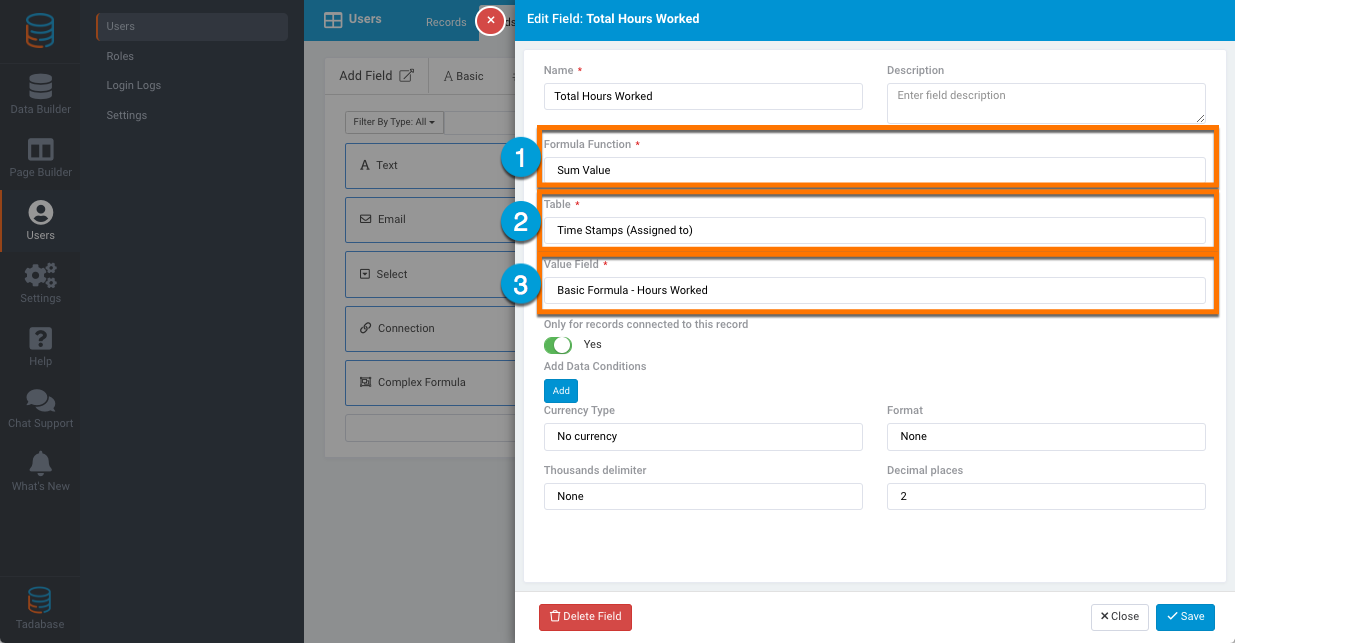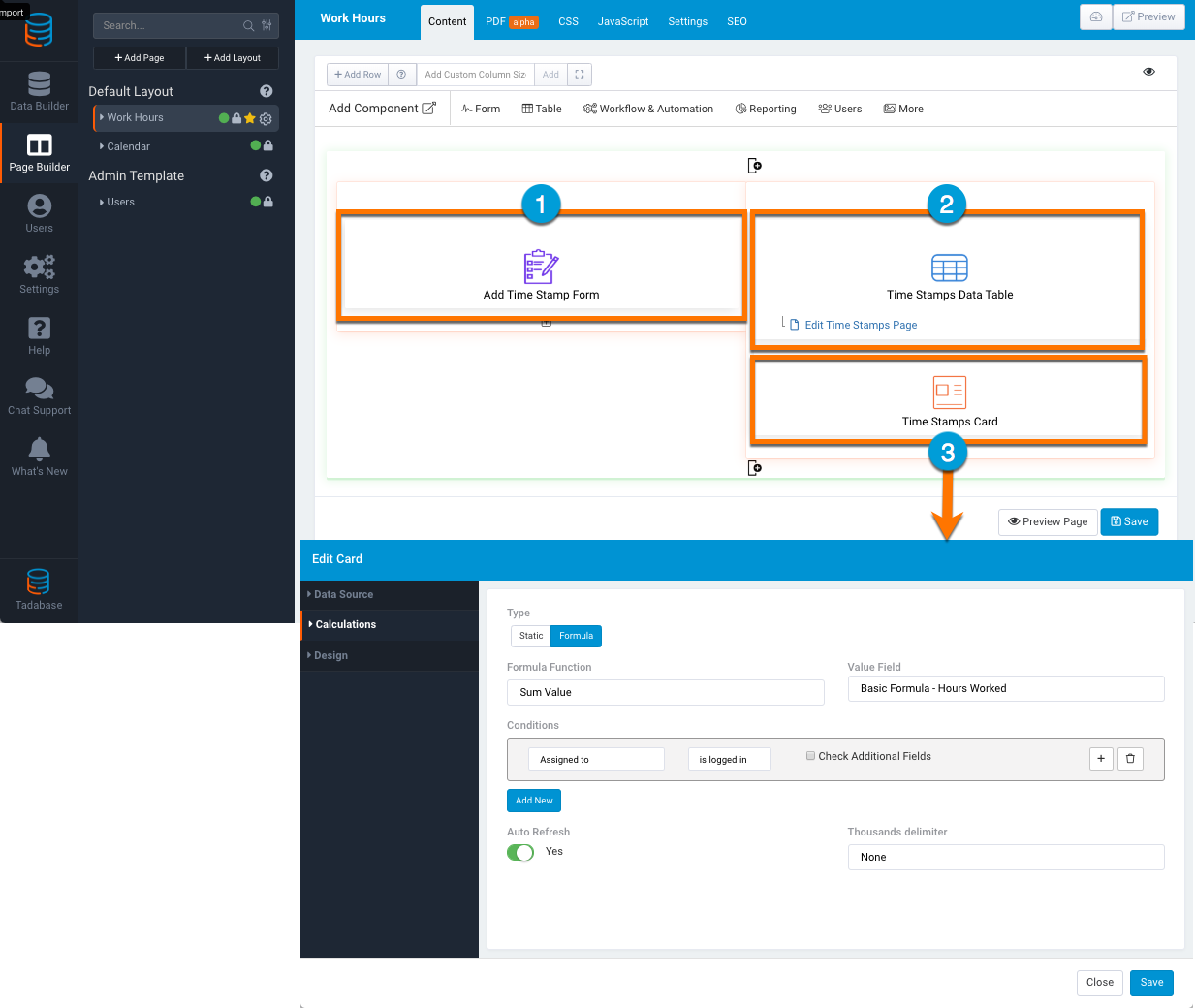# Sum date/time field

In this article, we will go over how to use the Date and Basic Formula field to sum all connected records with a complex formula.

We'll use an example to demonstrate this. In our example, we have a simple time tracking app where users can log in, enter a start and end time, and see the total time spent.

To get started, all we need is to add one table with 5 fields. In this example, we'll call the table Time Stamps.As you can see in the image above there are 5 fields added to the table.

1. Start Time (Date/Time Field)
2. End Time (Date/Time Field)
3. Total Hours Worked (Date Formula)
4. Basic Formula - Hours Worked (Basic Formula)
5. Assigned to (Connection)

1 & 2. Start Time & End Time (Date/Time Field)

These can be added by hovering over the Date option and then select Date & Time3. Total Hours Worked (Date Formula)

This field will be used to calculate the time spent on one record (End Time minus Start Time)4. Basic Formula - Hours Worked (Basic Formula)

This field is important because we need it to be able to SUM all records connected to the logged-in user.

We'll need to select the Date Formula field (in hours) as shown in the image below.5. Assigned to (Connection)

This is our connection field to the user's table (One Time Stamp to One User) so that we can know which timestamp belongs to which user.Now that we have our Time Stamps table all set up we can now head over to the default User's table and add a Complex (Sum) FormulaWe want this complex formula to sum the total amount of hours for each user so we'll confirm that (1) The formula Function is "Sum Value" (2) The table selected is "Time Stamps (Assigned to) and (3) The Value Field is "Basic Formula - Hours Worked"Now on our page builder, we can add (1) a form that will add a timestamp (2) a table that will display all of the user's timestamp and (3) a card component that will display the total amount of timeYou can also add (3) as an HTML component as shown in the image below.